0%

## Introduction

1. Definition

• Logical memory = a process’s memory
• As viewed (referenced) by a process
• Allocated without regard to physical memory
2. Problems with sharing memory

• Compiler generates memory reference
• Unknown where process will be located
• The protection problem

• Modifying another process’s memory
• The space problem

• The more processes there are, the less memory each individually can have
3. Logical vs. Physical Addressing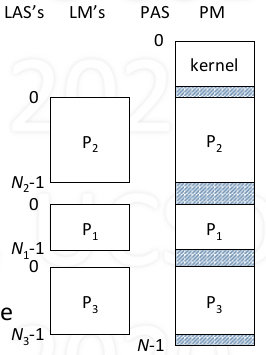• Assumes seperate memory starting at 0
• Compiler generated
• Independent of location in physical memory
• Convert logical to physical

• Via software: at load time
• Via hardware: at access time
• Hardware for Logical addressing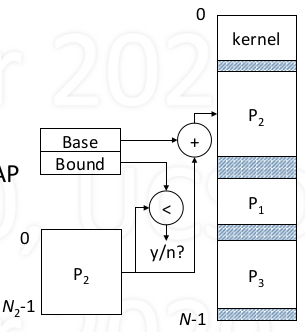• Base register filled with start address
• Achieves relocation
• To more process: change base
• Protection

• Bound register works with base register

• Achieves protection

• Memory Registers are part of context

• On every context switch

• Load base/bound register for selected process
• Kernel must be proetced from all processes
• Benefit

• Allows each proces to be seperated located
• Protecs each process from all others
4. Process mempory allocation

• Text: program instruction

• excute-only, fixed size
• Data: varaible (static, heap)

• dynamic allocation by request
• Stack: activation records, local variable

• Automatic growth/shrinkage
• Fitting process into memory

• Must find large enough hole
• May not succeed even if enought fragment space
• Even successul, it’s inefficient since space must be allocated for potential growth area
• Solution: break process into pieces

• Distribute into available holes
• Two approaches: Segment and Page

## Segementation

• Address space is a set of segments
• Segment: a linearly addressed memory

• Typically contains logically-related information
• Examples: program code, data, stack
• Each segment has an identifier s, and a size N

• s between 0 and S-1, S = max number of segments
• Logical addresses are of the form (s, i)

• offset i within segment s, i must be less than N
• Example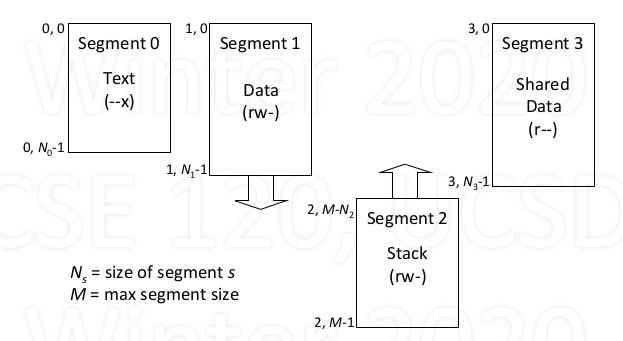• Problem: how to translate a logical address (s, i) into physical address a?
• Solution: use a segment (translate) table (ST)

• to segment s into base physical address b = ST(s)
• then add b and i
• physical address a = ST(s) + i

3. Segment Table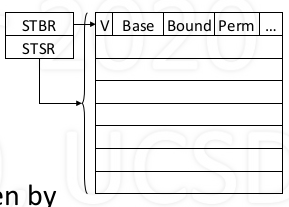• One table per process (typically)
• Table entry elements

• V: valid bit
• Base: segment location
• Bound: segment size
• Perm: permissions
• Location in memory given by

• Segment table base register(hardware)
• Segment table size register(hardware)

• physical address a = base of s + i
• do a series of checks

• s < STSR? -> is segment identifier valid or not?
• V == 1? -> the corresponding entry is valid?
• i < Bound? -> logical address is out of bound?
• Perm(op) -> that block has required operation(r/w/x)?
• Then access that physical address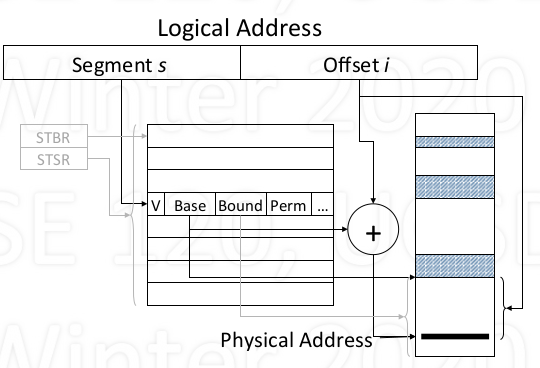4. Sizing the segment table

• Given 32 bit logical, 1 GB physical memory (max)

• 5 bit segment number, 27 bit offset

• Segement s: number of bits n specifies maxsize of table, where number of entries = $2^n$

• if 32 entries, n = 5
• Offset i: number of bits n specifies maxsize of segment

• 27 bits needed to size up to 128MB
• segment table

• V: 1 bit
• Base: number of bits needed to address physical memory

• 30 bits needed to address 1GB
• Bound: number of bits needed to specify max segment size

• 27 bits needed to size up to 128MB
• Perm: assume 3 bit (r/w/x)

• one entry: $1 + 30 + 27 + 3 + … = 61$+ bits $\approx$ 8 bytes

• whole table: 32 * 8 = 256 bytes
5. Pros and Cons

• Pros: Each segment can be

• located independently
• seperately protected
• grown/shrunk independently
• Segments can be shared by processes (via segment table)
• Cons: Variable-size allocation

• Difficult to find holes in physical memory
• External fragmentation

## Paging

1. Paged Address Space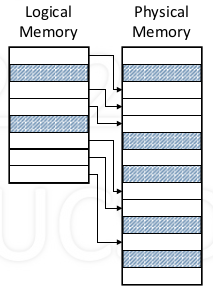• Logical (process) memory

• Linear sequence of pages
• Physical memory

• Linear sequence of frames
• Pages and frames

• Frame: a physical unit of information
• A page fits exactly into a frame
• Fixed size, all pages/frames same size

• Form of logical address: (p, i)

• p is page number, 0 to N - 1
• i is offset within page, since page size is fixed, i is guaranteed to be less than page size, no need to check
• Size of logical address space

• $N_L$ = max number of pages
• $N_L \times$ page size = size of logical address space

• Form of physical address: (f, i)

• f is frame number, 0 to N - 1
• i is offset within frame, less than frame size
• Size of physical address space

• $N_p$ = max number of frames
• $N_p \times$ frame size = size of physical address space

• Problem: how to translate logical address (p, i) into physical address (f, i)
• Solution: use a page (translation) table PT

• translate page p into frame f = PT(p)
• then concatenate f and i
• Physical address (f, i) = PT(p) || i = (PT(p), i)

5. Page table

• Each page of logical memory correspondings to entry in page table
• Page table maps logical page into frame of physical memory
• One table per process (typically)
• Table entry elements

• V: valid bit
• DPB: demand paging bits
• Frame: page location
• Location in memory given by

• Page table base register(PTBR) (hardware)
• Page table size register(PTSR) (hardware)

• Physical address = frame of p || offset i
• Do a series of checks (similar to segmenatation)

• p < PTSR?
• V == 1?
• Perm(op)?
6. Sizing the page table

• Given 32 bit logical, 1 GB physical memory (max)

• 20 bit page number, 12 bit offset

• page p: 20 bits to address $2^{20} =$ 1M entries
• offset i: 12 bits, page size = frame size = $2^{12} =$ 4096 bytes.
• Page table

• V: 1 bit
• DPB: 3 bits
• Frame: 18 bits to address $2^{30}/2^{12}$ frames
• Perm: 3bits
• One entry: $1+3+18+3+…= 25$+ bits $\approx$ 4 bytes
• Whole table size = 1M * 4 = 4 MB

1. Segments vs. Pages

• Segment is good “logical” unit of information

• Can be sized to fit any contents
• Makes sense to share (e.g., code, data)
• Can be protected according to contents
• Page is good “physical” unit of information

• Simple memory management
2. Combining segments and pages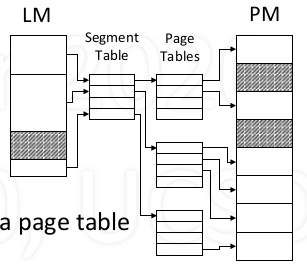• Logical memory

• composed of segments
• Each segment

• composed of pages
• Segment table

• Maps each segment to a page table
• Page tables

• Maps each page to physical page frames

• Logical address: [segment s, page p, offset i]
• Do various checks

• s < STSR, V == 1, p < bound, perm(op)
• May get a segmentation violation
• Use s to index segment table to get page table

• Use p to index page table to get frame f
• Physical address = concatenate (f, i)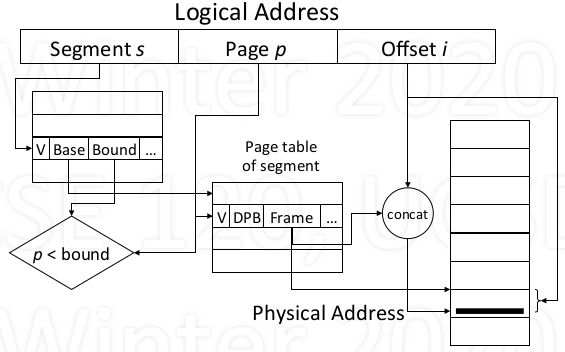1. Cost of translation

• Each lookup costs another memory reference

• For each reference, additional references required
• Slows machine down by factor of 2 or more
• Take advantage of locality of reference

• Most references are to a small number of pages
• Keep translation of these in high-speed memory
• Problem: don’t know which pages till accessed

2. Translation Look-aside Buffer (TLB)

• Fast memory keeps most recent translations
• If key matches, get frame number
• else wait for normal translation (in parallel)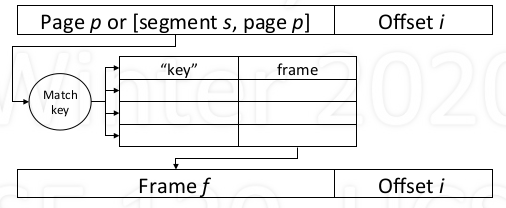3. Translation Cost with TLB

• Cost is determined by

• Speed of memory: ~100 nsec
• Speed of TLB: ~5 nsec
• Hit ratio: fraction of refs satisfied by TLB, ~99%
• Speed with no address translation: 100 nsec

• TLB miss: 200 nsec (100% slowdown)
• TLB hit: 105 nsec (5% slowdown)
• Average: 105 x 0.99 + 200 x 0.01 ~ 106 nsec
4. TLB Design Issues

• The larger the TLB

• the higher the hit rate
• the slower the reponse
• the greater the expense
• TLB has a major effect on performance!

• Must be flushed on context switched
• Alternative: tagging entries with PIDs